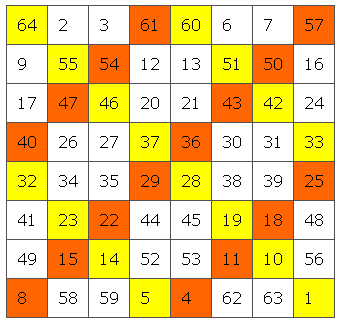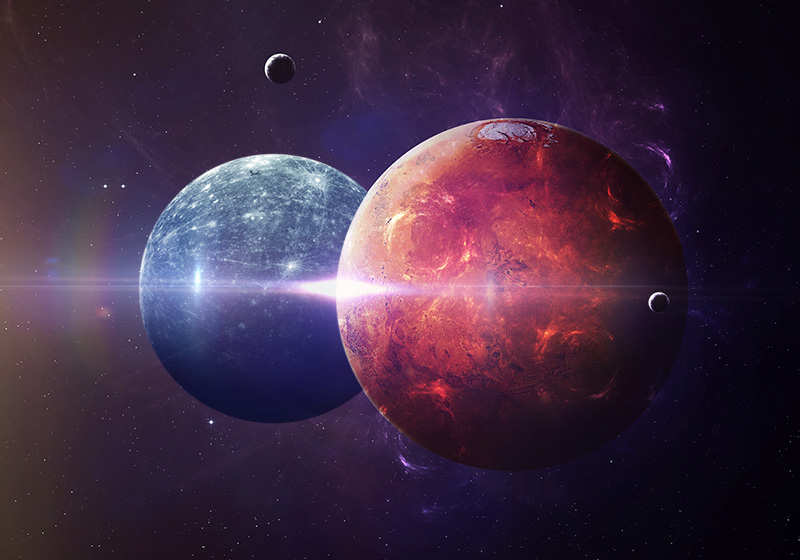# Auspicious Eight in Arithmetic, Geometry and Antiquity

Auspicious Eight in Arithmetic, Geometry and Antiquity.

Ancient Greek of the following mathematical operation:

1. Consider every odd number above one.
2. Square that number and add one to it.  As 5² + 1 = 25.
3. The resultant number will always be a multiple of 8 plus one.
4. Thus, 5² + 1 = (3 x 8) + 1.

Why was this important? Certain Greek philosophers knew: An advanced civilization (possibly Atlantis if you believe Plato), was once sustained by knowledge of number squares. That civilization enjoyed a Golden Age over an extended period of time. Today most view number squares as mere mathematical curiosities.The Great Pyramid in Egypt is also measured by a square of eight great cubits on each side. It partakes of the auspicious eight. A Great Cubit is 55 smaller Egyptian cubits of of 1.71818… feet. The perimeter measures 440 x 440 smaller Egyptian cubits.

## Characteristic Cousins are Found in Two Number Squares

Enjoy the above internal link from DSOworks.com. It  holds close to 600 posts on music, antiquity and number squares. At this point I am merely in the process of dividing the posts so that antiquity appears on this website and music on DSOworks. site. As I have the time, this website will grow. Currently, music has become quite pressing for me. Progress on the site will be slower.

### How 6 and 5 Join in the Auspicious Eight number square

On this square of numbers, any two oppositely positioned numbers total 65. Examples would be 64 + 1; or 55 + 10; or 15 + 50…Six was thought to represent yang. Five was thought to represent yin. The two come together in number 65. In this number square 32 sets of numbers come together to total 65. The Great Pyramid, among other things, brings the yin-yang relationship of 65 to the forefront. Another point on the Great Pyramid. The height is 5 Great Cubits x the length of one side opf the base as 8Also, if you check the internal link above, the 5 x 5 number square also holds a 65 order in it. Any row of five numbers totals 65.

### Mars and Mercury Are Characteristic Cousins by ancient  use of number Squares

Here’s what I find fascinating: The 8 x 8 number square was associated with Mercury. The 5 x 5 number square with Mars. They were in conjunction on June 28, 2017. Did these number squares harmonize with the Great Pyramid by common ratio numbers?Picture source published on June 28, 2017 Conjunction of Mercury and Mars written by Oranum Psychic Togro